ISSN ONLINE(2320-9801) PRINT (2320-9798)

All submissions of the EM system will be redirected to Online Manuscript Submission System. Authors are requested to submit articles directly to Online Manuscript Submission System of respective journal.

# Workflow Scheduling Using Heuristics Based Ant Colony Optimization

 1J.Elayaraja, 2 S.Dhanasekar PG Scholar, Department of CSE, Info Institute of Engineering, Coimbatore, India Assistant Professor, Department of CSE, Info Institute of Engineering, Coimbatore, India Related article at Pubmed, Scholar Google

Visit for more related articles at International Journal of Innovative Research in Computer and Communication Engineering

## Abstract

Ant Colony Optimization (ACO) is a Meta heuristic combinatorial optimization technique. Ant Colony System (ACS) is the algorithm in the ACO. This is based on the behavior of ants. Many ants starts to search food from the nest and travel some distance. In that time some ants goes to search food in some ways. At last all ants reach the destination point that way will be the shortest path. This is done by the pheromone updation. The leading ant deposits the pheromone while moving, the upcoming ants follows the leading ant by the chemical where it is high. This process is repeated until an optimal combination of ants solution is reached. Based on this concept we implement the algorithm to solve the real time problems like routing, assignment, scheduling

### Keywords

Ant Colony Optimization (ACO), grid computing, workflow scheduling.

### I. INTRODUCTION

The ant colony optimization process can be explained by representing the optimization problem as a multilayered graph as shown in Fig. 1 where the number of layers is equal to the number of design variables and the number of nodes in a particular layer is equal to the number of discrete values permitted for the corresponding design variable. Thus each node is associated with a permissible discrete value of a design variable. Figure 1 denotes a problem with six design variables with eight permissible discrete values for each design variable
The ACO process can be explained as follows. Let the colony consist of N ants. The ants start at the home node, travel through the various layers from the first layer to the last or final layer, and end at the destination node in each cycle or iteration. Each ant can select only one node in each layer. The nodes selected along the path visited by an ant represent a candidate solution. For example, a typical path visited by an ant is shown by thick lines in Fig. 1.1. This path represents the solution (x12, x23, x31, x45, x56, x64). Once the path is complete, the ant deposits some pheromone on the path. When all the ants complete their paths, the pheromones on the globally best path are updated using the global updating rule. In the beginning of the optimization process (i.e., in iteration 1), all the edges or rays are initialized with an equal amount of pheromone. As such, in iteration 1, all the ants start from the home node and end at the destination node by randomly selecting a node in each layer. The optimization process is terminated if either the prespecified maximum number of iterations is reached or no better solution is found in a prespecified number of successive cycles or iterations. The values of the design variables denoted by the nodes on the path with largest amount of pheromone are considered as the components of the optimum solution vector. In general, at the optimum solution, all ants travel along the same best (converged) path

### II. REVIEW OF ANT COLONY OPTIMIZATION

Ant Colony Optimization (ACO) is a metaheuristic combinatorial optimization technique that mimics the foraging behavior of Ants. As shown in Fig. 3, ACO starts with initialization of parameters and ants along with permissible range. Each ant and its permissible range are processed to construct path. The constructed path is explored to form different combination of possible discrete values for the decision variable and the objective function is evaluated. Check whether the optimum solution is reached or not. If yes stop the process otherwise the best path is chosen from the evaluated values and the pheromone updating is carried out on the best path to form new set of permissible ranges for the next iteration. Also at each iteration, new set of ants are generated randomly. The following issues are to be addressed while implementing ACO for any combinatorial optimization problem.

### III. ACO IMPLEMENTATION FOR SCHEDULING WORKFLOW

While implementing ACO for any scheduling algorithm, the following issues are to be addressed.
ï· Initialization of Pheromone
ï· Initialization of Heuristic information
ï· Random generation of Ants
ï· Mapping of Ant with path
ï· Evaluation of objective function
ï· Pheromone updating
The details of the above said issues are discussed in subsections as belowFigure 1 Graphical representation of ACO process in the form of multi-layered network
Working Principle of ACO
As shown in figure 1, ACO starts with initialization of parameters and ants along with permissible range. Each ant and its permissible range are processed to construct path. The constructed path is explored to form different combination of possible discrete values for the decision variable and the objective function is evaluated. Check whether the optimum solution is reached or not. If yes stop the process otherwise the best path is chosen from the evaluated values and the pheromone updation is carried out on the best path to form new set of permissible ranges for the next iteration. Also at each iteration, new set of ants are generated randomly. The following issues are to be addressed while implementing ACO for any combinatorial optimization problemFIG2:flow chart of ACO
Initialization of control Parameters
The most common parameters used in ACO algorithm are given below
ï§ Number of Variable - D
ï§ Number of Permissible Value - P
ï§ Number of Ants - N
ï§ Pheromone decay factor - ρ (lies bw 0 and 1)
ï§ Pheromone error factor - σ (lies bw 0 and 1)
ï§ Min. Pheromone value - ï´ 0 (lies bw 0 and 1)
ï§ Min. Pheromone value - ï´ 0 (lies bw 0 and 1)
ï§ Number of Iteration - I
Pheromone decay factor (ρ) is used to reduce the current pheromone value on each path after each ant tours using local pheromone updating rule. Minimum Pheromone value (ï´ 0 ) is used to find the minimum pheromone value among the all paths. Pheromone error factor (σ) is used to add some error while updating the pheromone in global pheromone updating rule.
A. Initialization of Pheromone
For each design variable, pheromone matrix is initialized. The number of pheromone value in a pheromone matrix depends on the number of permissible value ‘P’ for the design variable. For example if x1 is the design variable and it takes any value from 1 to 4 means, then the number of pheromone value is also 4 and each pheromone value is initialized to 1 in the pheromone matrix(1)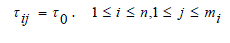(2)
(a) Calculation of probability value pij
For each design variable, probability matrix is constructed from the pheromone matrix by using the equation (4).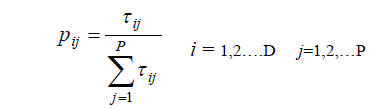The number of probability value in a probability matrix depends on the number of permissible value ‘P’ for the design variable
B. Calculation of Permissible Range/Path Construction
For each design variable, permissible range (PR)
matrix is constructed from the probability matrix by using the following procedure.
The number of permissible ranges for each design variable is equal to the number of permissible values of the design variable. Each permissible range represents the path to be explored by the ant for its food.
C. Random Generation of Ants
Ants for each design variable are randomly generated between the overall ranges of a permissible range matrix as given in Eq. (3). The number of ants for each design variable is taken arbitrarily depending on nature of the problem. To obtain better solution, colony size (number of ants) must be at least greater than or equal to the number of permissible range.(3)
Where,(4)
D. Mapping of Ant with Path / Path Identification
For each design variable, each ant value is mapped with its permissible range value for identifying the path. Once a path is identified, its corresponding index value is taken as candidate value for the design variable. The number of candidate value for each deign variable is equal to the number of ants.
E. Forming of Combinations
The candidate values of each design variable found during path identification are formed as different combination and are used for evaluating the objective function. If ‘N’ is the number of ants, and ‘D’ is the number of design variable then ‘ND’ combinations are formed.
F. Evaluation of Objective function
The combination value chosen for the design variables is used to evaluate the objective function. All such possible combinations are evaluated to give its objective valueG. Selection of best path
After evaluating the objective function, the value which gives optimum objective value is selected and its associated permissible range value is selected as best path and pheromone updation is carried out and the updated pheromone value is used to update the probability value which in turn will update the permissible range value.
H. Pheromone updation
The initial pheromone matrix is updated on the selected best path to direct future ants more strongly toward better solution. There are two kinds of pheromone updation. The local pheromone updating rule is given in equation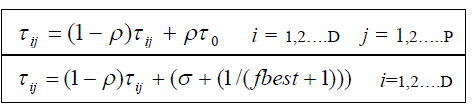The importance of global pheromone updation is, it distincts the best path with other paths by adding extra pheromone. It tends the future ants to select the path once again.
These steps will be repeated until we gets the accurate values for the problem.

### IV. SIMULATION RESULTS

This section presents the details of simulation carried out using a sample workflow that has two tasks. Seven instances are assigned to task1 and six instances are assigned to task2. The details of instances along with the values of QoS parameters such as time, cost and budget are shown in Table 1. The values are randomly generated by following the rule that for the same task, a service instance with higher reliability or shorter execution time may cost more money and vice versa.
TABLE I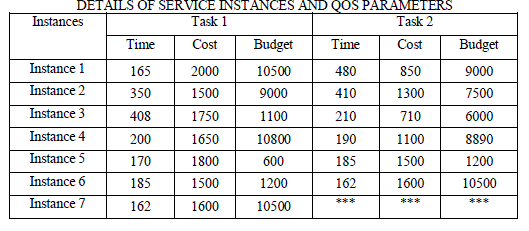In Eq. (1), the pheromone values are initialized using the maximum and minimum values of makespan taken from task 1 and task 2. The initial pheromone value is assigned to all service instances available in the both task and heuristics values are calculated for each service instance as discussed in Section .2..
This paper proposes four different heuristics in the algorithm including TG, CG, SB, and TC. Heuristics are also selected based on pheromone values. Heuristics selection of the ant followed by path constructed of the proposed ACO algorithm is pictorially shown in the Fig.3fig 3:procedure of an ant to build a solution
Experiments are conducted to compare the performance of these heuristics schemes and the results are shown in Fig. 4. The colony of size of this algorithm must be greater than or equal to the number of permissible path we have taken. The convergence of the optimal solution is much faster when increase the colony size in the algorithm.Fig. 4. Performance of different heuristics in case of makespan optimization
This paper has applied the user constraints budget and deadline in order to optimize the makespan. The optimal results were obtained with Colony Size: 30, Budget: 3300, and Deadline: 800.
Fig. 5 reveals the most attractive heuristic type in different stage of the algorithm. For example, in the makespan optimization under tight deadline constraints, at the beginning stage of the algorithm, heuristic 2 is able to find feasible solutions that satisfy the deadline constraints quickly. At later stages, the attraction of the heuristics selection has been changing due to the constraints. Finally, the cost heuristic takes hold in finding the optimal solution as shown in Fig. 4.Fig. 5. Heuristics type adopted by most ants
The convergence behavior of the proposed ACO is shown in Fig. 6. From Fig. 6, it is found that the proposed ACO has converged in the first five iterations itself.Fig. 6. Convergence of ACO
From the Table 2, it is found that, execution of instance 3 of task 1 followed instance 3 of task 2 is n optimal schedule of executing the chosen two task application in grid computing platform.
TABLE II:BEST SCHEDULE IN THE APPLICATION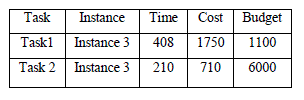### V. CONCLUSION

This paper has proposed ACO approach for scheduling workflows in computational grids. The proposed approach is implemented for a sample workflow application based on service oriented architecture. Two workflows with seven and six tasks are considered for simulation. QoS parameters considered in the simulation are time, cost, and budget and the optimization is based on user-defined QoS parameter. Heuristics considered in the simulation are Time, Cost, Budget and Total Cost and an adapted scheme is included to make the artificial ant to select heuristics based on pheromone value. Simulation results show the effectiveness of the proposed approach. In future, the same approach is planned to enhance it for more QoS parameters and Heuristics.

### REFERENCES

 Hamid Mohammadi Fard, Radu Prodan, and Thomas Fahringer, “A Truthful Dynamic Workflow Scheduling Mechanism for Commercial Multicloud Environments” IEEE Trans. on Parallel and Distributed Systems, Vol. 24, No. 6, June 2013.
 Wei-Neng Chen, Stud Mem, Jun Zhang “An Ant Colony Optimization Approach to a Grid Workflow Scheduling problem With Various Qos Requirements” IEEE Trans. on sys.,Man ,Cybern-Part C: Appl & Reviews, vol.39, no.1, Jan 2009.
 R. Buyya, D. Abramson, and J. Giddy, “A case for economy grid architecture for service oriented grid computing,” presented at the 10th Heterogeneous Comput. Workshop (HCW’ 2001), San Francisco, CA, Apr.
 F. Neubauer, A. Hoheisel, and J. Geiler, “Workflow-based grid applications,” Future Gen. Comput. Syst., vol. 22, pp. 6–15, 2006.
 Z. Shi and J. J. Dongarra, “Scheduling workflow applications on processors with different capabilities,” Future Gen. Comput. Syst., vol. 22, pp. 665– 675, 2006.
 L. Chunlin and L. Layuan, “QoS based resource scheduling by computational economy in computational grid,” Inf. Process. Lett., vol. 98, pp. 119– 126, 2006.
 H. XiaoShan and S. XiaoHe, “QoS guided min-min heuristic for grid task scheduling,” J. Comput. Sci. Technol., vol. 18, no. 4, pp. 442–451, 2003.
 M. M. Lopez, E. Heymann, and M. A. Senar, “Analysis of dynamic heuristics for workflow scheduling on grid systems,” in Proc. 5th Int.Symp. Parallel Distrib. Comput. (ISPDC’06), IEEE, Jul., pp. 199–207.
 M. Maheswaran et al., “Dynamic mapping of a class of independent tasks onto heterogeneous computing systems,” J. Parallel Distrib. Comput., vol. 59, pp. 107–131, 1999.
 H. Topcuoglu, S. Hariri, and M.-Y. Wu, “Performance-effective and low complexity task scheduling for heterogeneous computing,” IEEE Trans. Parallel Distrib. Syst., vol. 13, no. 3, pp. 260–274, Mar. 2002.
 L.Wang, H. J. Siegel, V. P. Roychowdhury, and A. A. Maciejewski, “Task matching and scheduling in heterogeneous computing environments using a genetic-algorithm-based approach,” J.Parallel Distrib. Comput., vol. 47, pp. 8–22, 1997.
 J.-K. Kim, et al., “Dynamically mapping tasks with priorities and multiple deadlines in a heterogeneous environment,” J. Parallel Distrib. Comput., vol. 67, pp. 154–169, 2007.
 S. Zheng,W. Shu, and L.Gao, “Task scheduling using parallel genetic simulated annealing algorithm,” in Proc. IEEE Int. Conf. Service Operations Logist. (SOLI’06), pp. 46–50.
 D. Merkle, M. Middendorf, and H. Schmeck, “Ant colony optimization for resource-constrained project scheduling,” IEEE Trans. Evol. Comput., vol. 6, no. 4, pp. 333–346, Aug. 2002.
 M. Dorigo, V. Maniezzo, and A. Colorni, “Ant system: optimization by a colony of cooperating agents,” IEEE Trans. Syst., Man, Cybern. B, Cybern., vol. 26, no. 1, pp. 29–41, Feb. 1996.
 M. Dorigo, V. Maniezzo, and A. Colorni, “Ant system: optimization by a colony of cooperating agents,” IEEE Trans. Syst., Man, Cybern. B,Cybern., vol. 26, no. 1, pp. 29–41, Feb. 1996.